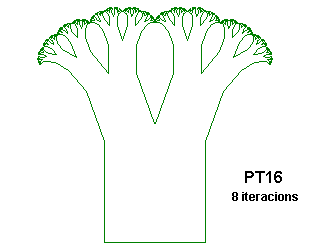ARBRES FRACTALS GENERATS COM A SISTEMES L

 PT1 {; Pythagorean Tree ; From "Chaos and Fractals" by Peitgen, Jürgens and Saupe ; Figure 2.63, p. 127 Angle 8 Axiom F++F[A]++F[B]++F A=-@IQ2F[A]++F[B]++F++F B=---@IQ2F++F[A]++F[B]++F }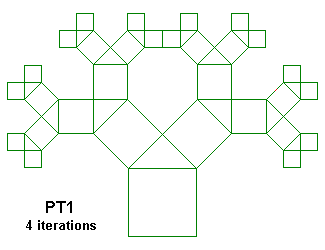PT2 {; Pythagorean Tree ; From "Chaos and Fractals" by Peitgen, Jürgens and Saupe ; Figures 2.64 and 2.65, p. 128 and 129 ; .866 is sqrt(3)/2 ; The two triangles are always attached in same orientation Angle 12 Axiom F+++F[A]+++F[B]+++F A=--@.5F[A]+++F[B]+++F+++F B=-----@.866F+++F[A]+++F[B]+++F }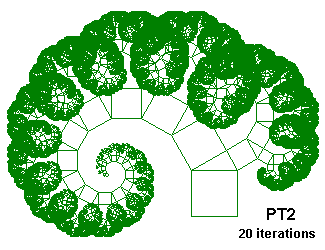PT3 {; Pythagorean Tree ; From "Chaos and Fractals" by Peitgen, Jürgens and Saupe ; Figures 2.64 and 2.65, p. 128 and 129 ; .866 is sqrt(3)/2 ; The two triangles flip their orientation after each step Angle 12 Axiom F+++F[A]+++F[B]+++F A=--@.5F[P]+++F[Q]+++F+++F B=-----@.866F+++F[P]+++F[Q]+++F P=-@.866F[A]+++F[B]+++F+++F Q=----@.5F+++F[A]+++F[B]+++F }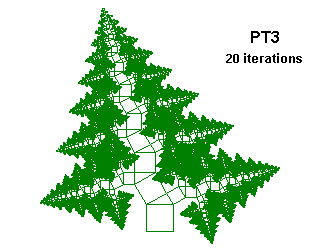PT4 {; Broccoli-Like Phythagoras Tree ; From "Chaos and Fractals" by Peitgen, Jürgens and Saupe ; Figure 2.67, p. 130 ; Using D, \nn and /nn ; .532 is 1/(2cos20°) Angle 3 ; Only to avoid errors, we do not use this angle Axiom D\90D[A]\90D[B]\90D A=/20@.532D[A]\90D[B]\90D\90D B=/160@.532D\90D[A]\90D[B]\90D }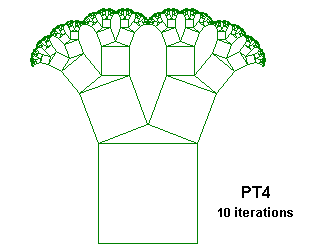PT5 {; Pythagorean Tree ; From "Chaos and Fractals" by Peitgen, Jürgens and Saupe ; Inspired on Figures 2.64 and 2.65, p. 128 and 129 ; Construction with a triangle of sides 3, 4 and 5 ; Using D, \nn and /nn, 53.13° is acos(3/5) Angle 3 ; Only to avoid errors, we do not use this angle Axiom D\90D[A]\90D[B]\90D A=/53.13@.6D[A]\90D[B]\90D\90D B=/143.13@.8D\90D[A]\90D[B]\90D }PT6 {; Pythagorean Tree ; From "Chaos and Fractals" by Peitgen, Jürgens and Saupe ; Inspired on Figures 2.64 and 2.65, p. 128 and 129 ; Construction with a triangle of sides 3, 4 and 5 ; Using D, \nn and /nn, 53.13° is acos(3/5) Angle 3 ; Only to avoid errors, we do not use this angle Axiom D\90D[A]\90D[B]\90D A=/53.13@.6D[P]\90D[Q]\90D\90D B=/143.13@.8D\90D[P]\90D[Q]\90D P=/36.87@.8D[A]\90D[B]\90D\90D Q=/126.87@.6D\90D[A]\90D[B]\90D }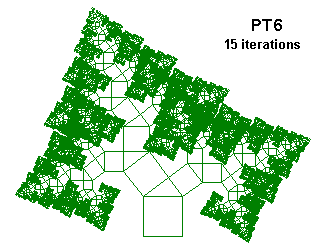PT7 {; Pythagorean Tree ; From "Chaos and Fractals" by Peitgen, Jürgens and Saupe ; Inspired on Figures 2.64 and 2.65, p. 128 and 129 ; Construction with a triangle of sides 5, 12 and 13 ; Using D, \nn and /nn, 67.38° is acos(5/13) Angle 3 ; Only to avoid errors, we do not use this angle Axiom D\90D[A]\90D[B]\90D A=/67.38@.3846D[A]\90D[B]\90D\90D B=/157.38@.9231D\90D[A]\90D[B]\90D }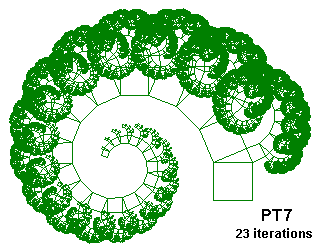PT8 {; Pythagorean Tree ; From "Chaos and Fractals" by Peitgen, Jürgens and Saupe ; Inspired on Figures 2.64 and 2.65, p. 128 and 129 ; Construction with a triangle of angles 40°, 50° and 90° ; Using D, \nn and /nn, .643 is cos50° Angle 3 ; Only to avoid errors, we do not use this angle Axiom D\90D[A]\90D[B]\90D A=/50@.643D[A]\90D[B]\90D\90D B=/140@.766D\90D[A]\90D[B]\90D }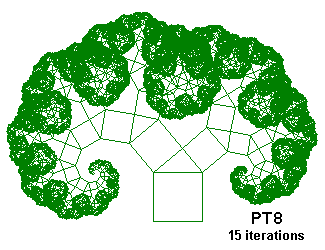PT9 {; Phytagorean Tree constructed over a rectangle ; From "Chaos and Fractals" by Peitgen, Jürgens and Saupe ; Inspired on Figures 2.64 and 2.65, p. 128 and 129 ; .866 is sqrt(3)/2 Angle 12 Axiom F+++FF[A]+++F[B]+++FF A=--@.5FF[A]+++F[B]+++FF+++F B=-----@.866F+++FF[A]+++F[B]+++FF }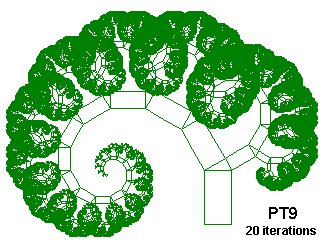PT10 {; Broccoli-Like Phythagoras Tree over a rectangle ; From "Chaos and Fractals" by Peitgen, Jürgens and Saupe ; Inspired on Figure 2.67, p. 130 ; Using D, \nn and /nn ; .532 is 1/(2cos20°) Angle 3 ; Only for to avoid errors, we not use this angle Axiom DD\90D[A]\90DD[B]\90D A=/20@.532D[A]\90DD[B]\90D\90DD B=/160@.532DD\90D[A]\90DD[B]\90D }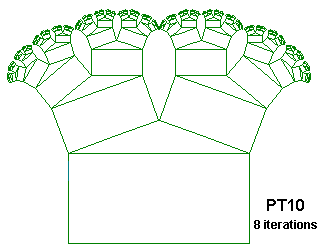PT11 {; Phytagorean Tree ; From "Chaos and Fractals" by Peitgen, Jürgens and Saupe ; Inspired on Figures 2.64 and 2.65, p. 128 and 129 ; .866 is sqrt(3)/2 ; The triangle on the right flips his orientation after each step ; The triangle on the left is always attached in the same orientation Angle 12 Axiom F+++F[A]+++F[B]+++F A=--@.5F[P]+++F[Q]+++F+++F B=-----@.866F+++F[A]+++F[B]+++F P=-@.866F[A]+++F[B]+++F+++F Q=----@.5F+++F[A]+++F[B]+++F }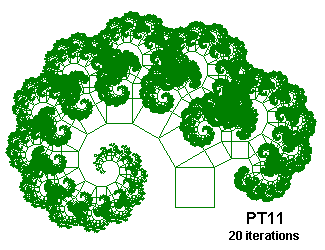PT12 {; Phytagorean Tree ; From "Chaos and Fractals" by Peitgen, Jürgens and Saupe ; Inspired on Figures 2.64 and 2.65, p. 128 and 129 ; .866 is sqrt(3)/2 ; The triangle on the left flips his orientation after each step ; The triangle on the right is always attached in the same orientation Angle 12 Axiom F+++F[A]+++F[B]+++F A=--@.5F[A]+++F[B]+++F+++F B=-----@.866F+++F[P]+++F[Q]+++F P=-@.866F[A]+++F[B]+++F+++F Q=----@.5F+++F[A]+++F[B]+++F }PT13 {; Phytagorean Tree, using G ; From "Chaos and Fractals" by Peitgen, Jürgens and Saupe ; Inspired on Figures 2.64 and 2.65, p. 128 and 129 ; .866 is sqrt(3)/2 Angle 12 Axiom F+++F[A]+++G[B]+++F A=--@.5F[A]+++G[B]+++F+++G B=-----@.866G+++F[A]+++G[B]+++F }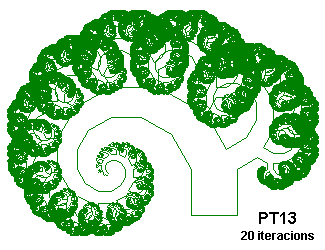PT14 {; Phytagorean Tree, using G and over a rectangle ; From "Chaos and Fractals" by Peitgen, Jürgens and Saupe ; Inspired on Figures 2.64 and 2.65, p. 128 and 129 ; .866 is sqrt(3)/2 Angle 12 Axiom F+++FF[A]+++G[B]+++FF A=--@.5FF[A]+++G[B]+++FF+++G B=-----@.866G+++FF[A]+++G[B]+++FF }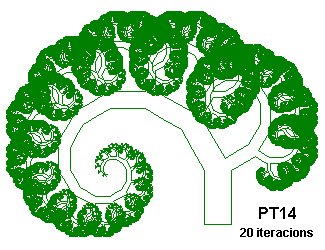PT15 {; Phytagorean Tree, using G ; From "Chaos and Fractals" by Peitgen, Jürgens and Saupe ; Inspired on Figures 2.64 and 2.65, p. 128 and 129 ; .866 is sqrt(3)/2 Angle 12 Axiom F+++F[A]+++G[B]+++F A=--@.5F[P]+++G[Q]+++F+++G B=-----@.866G+++F[P]+++G[Q]+++F P=-@.866F[A]+++G[B]+++F+++G Q=----@.5G+++F[A]+++G[B]+++F }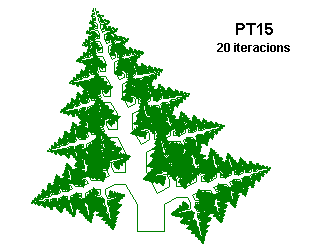PT16 {; Broccoli-Like Phythagoras Tree, using D ; From "Chaos and Fractals" by Peitgen, Jürgens and Saupe ; Inspired on Figure 2.67, p. 130 ; .532 is 1/2(cos20º) Angle 3 ; Only for to avoid errors, we not use this angle Axiom D\90D[A]\90M[B]\90D A=/20@.532D[A]\90M[B]\90D\90M B=/160@.532M\90D[A]\90M[B]\90D }